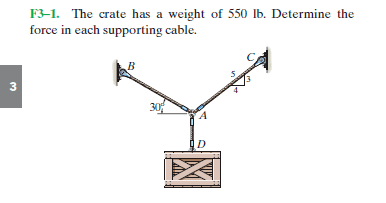# Free body diagram help

## Homework Statement## The Attempt at a Solution

I got the components of each force. I called the Force from BA "B" and the force from CA "C" and then the force going downward on D "W"

FBy=sin30*B
FBx=-cos30*B

FCy=3/5*C
FCx=4/5*C

W=-500
~~~~~~
ΣFx= -B*cos30 + 4/5C = 0
ΣFy= B*sin30 + 3/5C - 500 = 0

-0.866*B +0.8C=0
0.5*B+0.6C=500

Now when I solve the system of equations by elimination I'm getting B=434 and C=470 (I even checked on a calculator) , but the back of the book says 478 for B and 518 for C

What am I doing wrong?

I know this is kind of a dumb question like it's basic precalc but I've done it so many times over and over again I don't know what im going wrong